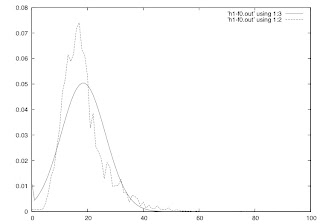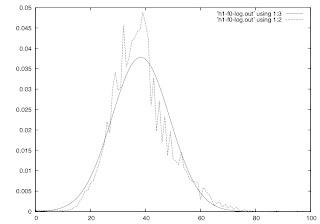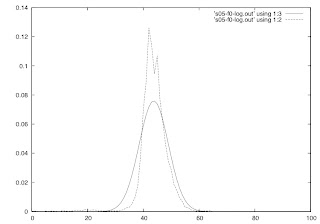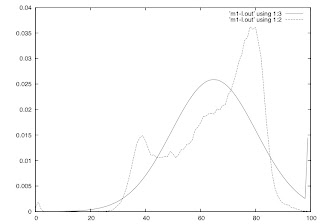Friday, August 06, 2010

Z-score normalization of pitch and intensity.

You may have noticed a post that was up here a few weeks ago about z-score normalization of pitch.  Well, my calculation of KL-divergence had a bug in it, and the conclusions of the post were wrong.  So I deleted it and am replacing it with this.

So the question is this.  When normalizing pitch and intensity values across speakers, what is the best approach.  Z-score normalization has a number of attractive properties -- it's relatively robust to outliers, it can be efficiently calculated -- however, it assumes a that the values being normalized follow a normal distribution.  Specifically z-score normalized values are calculated as follows:

$x^* = \frac{x-\mu}{\sigma}$

Where $\mu$ is the mean, and $\sigma$ the standard deviation of the known population of values.  So, the normalized value $x^*$ is how far the initial value $x$ was from the mean $\mu$ expressed in terms of a number of standard deviations.

So the question is when speaker normalizing pitch and intensity, is it better to use pitch or log pitch?  intensity or log intensity (or exp intensity, for that matter)?  The intuition about investigating these variations on Hz and dB come from perceptual qualities.  Human hearing perceives pitch and loudness on a logarithmic scale.  While this doesn't mean that the two qualities follow a log-normal distribution, processing logarithmic units gives some explanatory advantage.

To answer this question I calculated the KL divergence between the histogram of pitch and intensity values (with 100 equally spaced bins) and the maximum likelihood estimate Gaussian distribution.  I did this for the 4 speakers in the Boston Directions Corpus (BDC), the 6 speakers in the Boston University Radio News Corpus (BURNC), and 4 speakers of Mandarin Chinese speaking English.  This was repeated for log pitch and log intensity and exponentiated pitch and intensity.

The pitch results are mixed.  For 7 of 10 native American English speakers, log-pitch is more gaussian than pitch.  For the remaining three, the raw pitch is more gaussian.  (The exponentiated pitch values are never better.)  On average, the KL-divergence of log-pitch is lower (.1455 vs. .1683).  While not decisive, it is better to perform z-score normalization of log-pitch on American English speech. Here are some histograms and normal distributions from speaker h1 from the BDC.raw pitchlog pitch

But something interesting happens with the native Mandarin speakers.  Here, the raw pitch (.271) has a more gaussian distribution than log pitch (.397).  This could be a recording condition effect, but there could be an influence of Chinese being a tonal language that is coming into play here.  I'll be looking into this more...Mandarin Raw pitchMandarin log pitch

For intensity, the results are clear.  It's better to z-score normalize raw intensity (dB) than log intensity.  Across all speakers (but one -- h3 from BDC) raw intensity (.154) is more gaussian than log intensity (.230).  But intensity (dB) is already a logarithmic unit.  So we compared raw intensity with exponentiated intensity values, we found that exp intensity is slightly more gaussian than raw intensity (.143).  I'm skeptical that normalizing by exp intensity will have a dramatic effect, but it's better on all speakers but one.raw intensityexp intensitylog intensity

However, the intensity distributions look pretty bimodal or maybe even trimodal.  It might be better to normalize with a mixture of gaussians rather than a standard z-score.  Something to keep in mind.

Aside: About speaker h3 from the BDC.  The intensity values from this speaker show that the log intensity is most gaussian (.346), more than raw intensity (.696) while the exp intensity is the least (1.16).  I don't yet have a good explanation for what makes this speaker different, but it's worth noting that even when trying to normalize out speaker differences, the normalization routine can have inconsistencies across speakers.